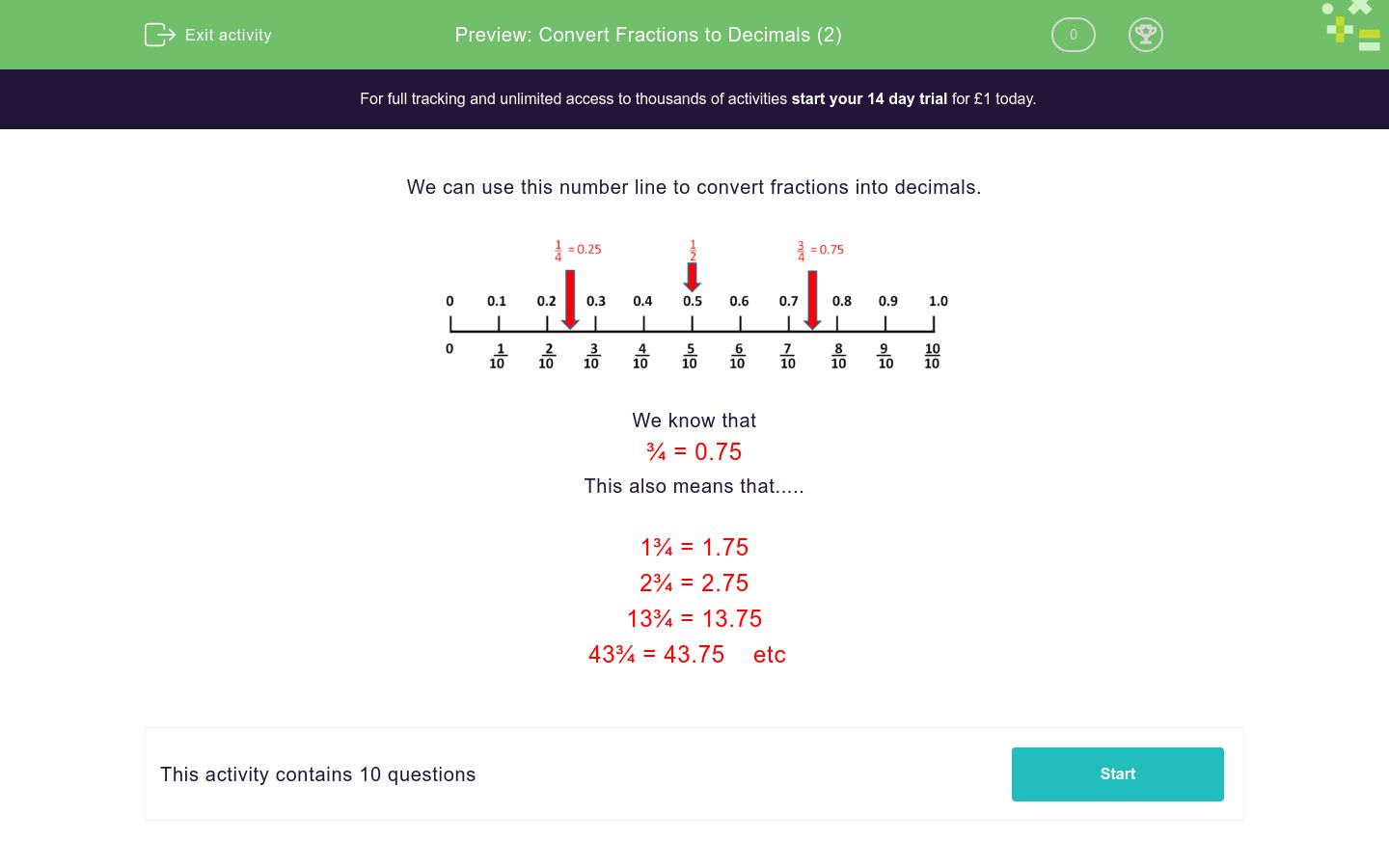# Convert Fractions to Decimals (2)

In this worksheet, students convert simple mixed numbers into decimals.Key stage:  KS 2

Curriculum topic:   Number: Fractions and Decimals

Curriculum subtopic:   Recognise Decimal Equivalents

Difficulty level:### QUESTION 1 of 10

We can use this number line to convert fractions into decimals.We know that

¾ = 0.75

This also means that.....

1¾ = 1.75

2¾ = 2.75

13¾ = 13.75

43¾ = 43.75    etc

Use the number line to help you convert this fraction into a decimal amount.Use the number line to help you convert this fraction into a decimal amount.Use the number line to help you convert this fraction into a decimal amount.Use the number line to help you convert this fraction into a decimal amount.Use the number line to help you convert this fraction into a decimal amount.

10¼Use the number line to help you convert this fraction into a decimal amount.

14¼Use the number line to help you convert this fraction into a decimal amount.

30½Use the number line to help you convert this fraction into a decimal amount.

30¾Use the number line to help you convert this fraction into a decimal amount.

25¼Use the number line to help you convert this fraction into a decimal amount.

75¾• Question 1

Use the number line to help you convert this fraction into a decimal amount.7.7
• Question 2

Use the number line to help you convert this fraction into a decimal amount.37.9
• Question 3

Use the number line to help you convert this fraction into a decimal amount.40.3
• Question 4

Use the number line to help you convert this fraction into a decimal amount.99.9
• Question 5

Use the number line to help you convert this fraction into a decimal amount.

10¼10.25
• Question 6

Use the number line to help you convert this fraction into a decimal amount.

14¼14.25
• Question 7

Use the number line to help you convert this fraction into a decimal amount.

30½30.5
• Question 8

Use the number line to help you convert this fraction into a decimal amount.

30¾30.75
• Question 9

Use the number line to help you convert this fraction into a decimal amount.

25¼25.25
• Question 10

Use the number line to help you convert this fraction into a decimal amount.

75¾75.75
---- OR ----

Sign up for a £1 trial so you can track and measure your child's progress on this activity.

### What is EdPlace?

We're your National Curriculum aligned online education content provider helping each child succeed in English, maths and science from year 1 to GCSE. With an EdPlace account you’ll be able to track and measure progress, helping each child achieve their best. We build confidence and attainment by personalising each child’s learning at a level that suits them.

Get started